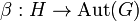# Tensor product of groups is commutative up to natural isomorphism

## Statement

Suppose$G$ and$H$ are (not necessarily abelian) groups with a compatible pair of actions$\alpha:G \to \operatorname{Aut}(H)$ and$\beta:H \to \operatorname{Aut}(G)$. We can define the tensor product of groups$G \otimes H$ and also the tensor product of groups$H \otimes G$. The claim is that these tensor products are isomorphic groups with a natural isomorphism$G \otimes H \to H \otimes G$ given as follows on a generating set:$g \otimes h \mapsto (h \otimes g)^{-1}$

Note that this natural isomorphism is self-inverse, i.e., the natural isomorphism from$G \otimes H$ to$H \otimes G$ is the inverse map to the similarly defined natural isomorphism from$H \otimes G$ to$G \otimes H$.

## Proof

### Proof idea

Consider the two axioms that define the tensor product:

•$(g_1g_2) \otimes h = ((g_1 \cdot g_2) \otimes (g_1 \cdot h))(g_1 \otimes h)$
•$g \otimes (h_1h_2) = (g \otimes h_1)((h_1 \cdot g) \otimes (h_1 \cdot h_2))$

Note that apart from interchanging the roles of$G$ and$H$, the other key difference between the two axioms is that the order of multiplication on the respective right sides differs. Since the inverse map is involutive, this explains why$g \otimes h \mapsto (h \otimes g)^{-1}$ works.